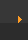## Chemical Bonding: Lewis Structure for O2

### Drawing the Lewis Structure for O3

Viewing Notes:

• For the Lewis Structure for O3 it is possible to draw it two different says (slightly different, but still important). These are called resonance structures.
• O3 (Ozone) is important in the upper atomsphere since it blocks UV light that can be harmful to humans (for example: causing skin cancer).
• Be sure that you don't use more than the 18 valence electrons available.

Transcript: Hi, this is Dr. B. Let's draw the Lewis structure of O3 or ozone. Start out by looking at Oxygen on the periodic table. It's in group 6 or 16, has 6 valence electrons, but we have three of them so let's multiply that by 3 to give us a total of 18 valence electrons. That's how many we have to work with. Draw our three Oxygens right here, and, so we have 18 valence electrons. Let's start by putting two between each of the Oxygens there, that forms a chemical bond. So we have 2, 4, 6, 8, 10, 12, 14, 16, 18.

Let's check now and see if we have octets. Over here, 8; over here, as well, so those have octets. But in the center, this Oxygen here only has six. So we need two more valence electrons. What we can do is take two from the outside and share them. When we do that, we form a double bond. And, let's see, now we have 2, 4, 6, 8 there. We have 8 for the center, and then we have 8 over here. So we've fulfilled the octet rule for each of the Oxygens.

The only problem is, we could've had the double bond over here and the single bond here. Both are correct. When you have structures when you can draw it two different ways, those are called resonance structures. We end up with something like this right here where we draw it both ways and we put an arrow between to show that it could be either. You could have a single here and a double here, or a double here and a single here. It's really the same thing.

This is Dr. B., and thanks for watching.

 Search our 100+ Lewis Structures   (Opens New Window) See the Big List of Lewis Structures Frequently Tested Lewis Structures Basic CH4,   NH3,   C2H4,   O2,   N2 Intermediate O3,   BBr3,   I3-,   BrF5,   NO Advanced SO3,   H2SO4,   OCN-,   XeO3,   ClO4-